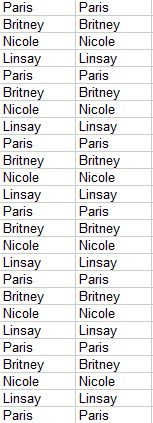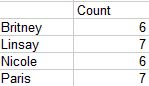## Single column manipulation using query function

This post is in continuation of my earlier post on query function. for basic understand the query function have a look at the following link https://anandexcels.wordpress.com/2013/11/01/query-function-in-google-sheets/

we will continue to use my earlier example only.

suppose if we want to count the First name and list them.

we can do this if we have multiple columns of data, suppose we have only one column we want to count as well as list them. you cannot do that using query function alone. we have to manipulate data using the Index function.

the formula for this is

=index(if({1,1},B2:B27))

this will create an array 2 X  26 on flyNow we have two column of data we can use the query function to manipulate this

=query(index(if({1,1},B2:B27));”select Col1,count(Col2) where Col1 <>” group by Col1 label count(Col2) ‘Count’ “)

the result will beObservations:

(1) We have not used the A, B instead we have used Col1 and Col2

(2) because the data is generated using the index function on fly

(3) we can create the copy of existing data using array constants

(4) the logic is within {1,1}

(5) you must use comma (in our example the data is single column), you should not use semi-colon

(6) comma is used for column manipulation and semi-colon is used for row manipulation

(7) this undocumented rules of array